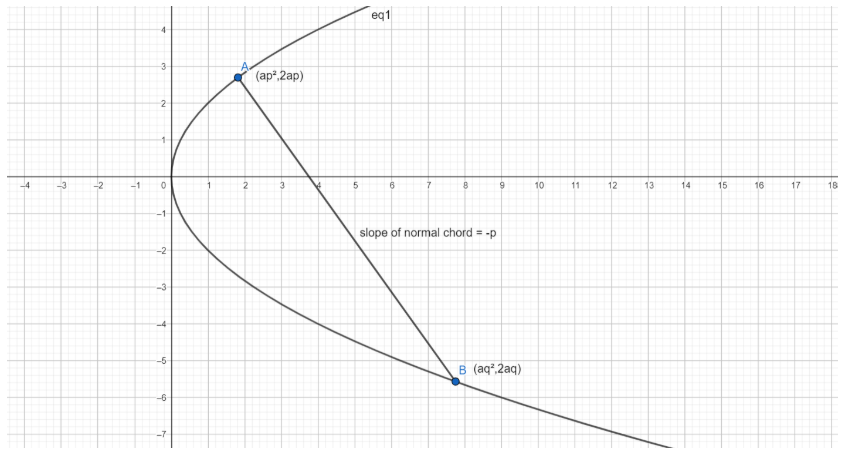QUESTION

# Prove that the locus of the centre of the circle , which passes through the vertex of a parabola and through its intersections with a normal chord, is the parabola $2{{y}^{2}}=ax-{{a}^{2}}$.

Hint: First, find the circle by assuming a general equation first, and then substituting for the $x$ and $y$ coordinates of the centre. Assume the parabola to have the general equation ${{y}^{2}}=4ax$, and then start working with a normal chord. The property of a normal chord is that the parameters $(t)$ of the two points where it cuts the parabola are related as : ${{t}_{1}}+{{t}_{2}}=-\dfrac{2}{{{t}_{1}}}$, if the parameter of the point which the normal is drawn to actually is ${{t}_{1}}$, or it’s represented as $(a{{t}_{1}}^{2},2a{{t}_{1}})$. The parameter of the point where this normal again cuts the parabola (not necessarily normally) is ${{t}_{2}}$.

Complete step-by-step solution -

Let the required circle be : ${{x}^{2}}+{{y}^{2}}-2hx-2ky+c=0$ ……….(1)
Where, the centre of the circle = $(h,k)$.
Since the first condition of the circle says that it has to pass through the vertex of the parabola ${{y}^{2}}=4ax$, we need to make the vertex satisfy equation (1) and simplify the equation we assumed till we reach the answer.
The vertex of the parabola ${{y}^{2}}=4ax$ will be $(0,0)$ since the vertex of a parabola with the general form ${{y}^{2}}=4ax$ is the same, and as we can see here, the parabola we have assumed is the general form itself.
Thus, the vertex $(0,0)$ will have to satisfy (1).
Substituting the coordinates of the vertex in (1), we get :
\begin{align} & {{x}^{2}}+{{y}^{2}}-2hx-2ky+c=0 \\ & \Rightarrow 0+0-2h0-2k0+c=0 \\ & \Rightarrow c=0 \\ \end{align}
Thus, we have a simplified equation which is : ${{x}^{2}}+{{y}^{2}}-2hx-2ky=0$ ……(2)
Moving ahead, we come to the concept of a normal chord.
In a parabola, when the normal is dropped on one point, it cuts the parabola at another point, the chord thus obtained is called a normal chord.
Let the normal be dropped on a point $(at_{1}^{2},2a{{t}_{1}})$, and let it cut the parabola again at a point $(at_{2}^{2},2a{{t}_{2}})$. Then, we have a result regarding these two points :
This result states that the parameters of the two points are related as :
${{t}_{1}}+{{t}_{2}}=-\dfrac{2}{{{t}_{1}}}$ …………………………(3)
and this result holds for any combination of ${{t}_{1}}$ and ${{t}_{2}}$.
Since in the question, it is given that the circle has to pass through the intersections of the normal chord with the parabola, it means that both the points $(at_{1}^{2},2a{{t}_{1}})$ and $(at_{2}^{2},2a{{t}_{2}})$ will have to satisfy the equation of the circle we need to find, or equation (2).
Substituting for $(at_{1}^{2},2a{{t}_{1}})$ first, we get :
\begin{align} & {{x}^{2}}+{{y}^{2}}-2hx-2ky=0 \\ & \Rightarrow {{(at_{1}^{2})}^{2}}+{{(2a{{t}_{1}})}^{2}}-2h(at_{1}^{2})-2k(2a{{t}_{1}})=0 \\ & \Rightarrow {{a}^{2}}t_{1}^{4}+4{{a}^{2}}t_{1}^{2}-2hat_{1}^{2}-4ak{{t}_{1}}=0 \\ & \Rightarrow at_{1}^{3}+4a{{t}_{1}}-2h{{t}_{1}}-4k=0 \\ & \Rightarrow at_{1}^{3}+{{t}_{1}}(4a-2h)-4k=0 \\ \end{align}
Let’s call the final equation, as equation (4).
Now, substituting $(at_{2}^{2},2a{{t}_{2}})$, we get :
\begin{align} & {{x}^{2}}+{{y}^{2}}-2hx-2ky=0 \\ & \Rightarrow {{(at_{2}^{2})}^{2}}+{{(2a{{t}_{2}})}^{2}}-2h(at_{2}^{2})-2k(2a{{t}_{2}})=0 \\ & \Rightarrow {{a}^{2}}t_{2}^{4}+4{{a}^{2}}t_{2}^{2}-2hat_{2}^{2}-4ak{{t}_{2}}=0 \\ & \Rightarrow at_{2}^{3}+4a{{t}_{2}}-2h{{t}_{2}}-4k=0 \\ & \Rightarrow at_{2}^{3}+{{t}_{2}}(4a-2h)-4k=0 \\ \end{align}
Let’s call this equation as equation (5).
Subtracting (5) from (4), we get :
\begin{align} & at_{1}^{3}-at_{2}^{3}+(4a-2h){{t}_{1}}-(4a-2h){{t}_{2}}-4k+4k=0 \\ & \Rightarrow a(t_{1}^{3}-t_{2}^{3})+(4a-2h)({{t}_{1}}-{{t}_{2}})=0 \\ & \Rightarrow a({{t}_{1}}-{{t}_{2}})(t_{1}^{2}+t_{2}^{2}+{{t}_{1}}{{t}_{2}})+(4a-2h)({{t}_{1}}-{{t}_{2}})=0 \\ & \Rightarrow (t_{1}^{2}+t_{2}^{2}+{{t}_{1}}{{t}_{2}})=\dfrac{2h-4a}{a} \\ \end{align}
Let’s call the equation obtained above as equation (6)
From equation (3), we can reduce the already obtained equation to be :
\begin{align} & {{t}_{1}}+{{t}_{2}}=-\dfrac{2}{{{t}_{1}}} \\ & \Rightarrow t_{1}^{2}+{{t}_{1}}{{t}_{2}}=-2 \\ \end{align} ……………………(7)

Subtracting (7) from (6), we get :
\begin{align} & t_{2}^{2}=\dfrac{2h-4a}{a}+2 \\ & \Rightarrow t_{2}^{2}=\dfrac{2h-2a}{a} \\ & \Rightarrow {{t}_{2}}=\sqrt{\dfrac{2h-2a}{a}} \\ \end{align}

Substituting the value of ${{t}_{2}}$ thus obtained in equation (5), we get :
\begin{align} & at_{2}^{3}+(4a-2h){{t}_{2}}-4k=0 \\ & \Rightarrow {{t}_{2}}(at_{2}^{2}+4a-2h)=4k \\ & \Rightarrow \sqrt{\dfrac{2h-2a}{a}}(a(\dfrac{2h-2a}{a})+4a-2h)=4k \\ & \Rightarrow \sqrt{\dfrac{2h-2a}{a}}(2h-2a+4a-2h)=4k \\ & \Rightarrow \sqrt{\dfrac{2h-2a}{a}}(-2a)=4k \\ & \Rightarrow \sqrt{\dfrac{2h-2a}{a}}=-\dfrac{2k}{a} \\ \end{align}
Squaring both sides, we get :
\begin{align} & \Rightarrow \dfrac{2h-2a}{a}=\dfrac{4{{k}^{2}}}{{{a}^{2}}} \\ & \Rightarrow a(2h-2a)=4{{k}^{2}} \\ & \Rightarrow a(h-a)=2{{k}^{2}} \\ & \Rightarrow 2{{k}^{2}}=a(h-a) \\ \end{align}
This equation shows a relation between the $x$ and $y$ coordinates of the centre of the circle, which follows the conditions mentioned in the question. The last step is to replace $(h,k)$ with $(x,y)$. Doing so we get :
\begin{align} & 2{{k}^{2}}=a(h-a) \\ & \Rightarrow 2{{y}^{2}}=ax-{{a}^{2}} \\ \end{align}
Which matches the equation mentioned in the question. Hence proved, this is the required equation.
The equation mentioned in the question is indeed the equation of the parabola that cuts the general parabola at the vertex and at the points of intersection of the normal chord with the general parabola.

Note: Here is a diagram to illustrate the concept of normal chords :Here, the parameters of point $A$ and $B$ are $p$ and $q$ respectively. Since chord $AB$ is a normal chord, its slope = $-p$, and the result relating $p$ and $q$ also holds true.